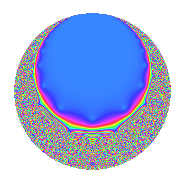# Properties

 Label 4.12.aLevel 4 Weight 12 Character orbit a Rep. character $$\chi_{4}(1,\cdot)$$ Character field $$\Q$$ Dimension 1 Newform subspaces 1 Sturm bound 6 Trace bound 0

# Related objects

## Defining parameters

 Level: $$N$$ = $$4 = 2^{2}$$ Weight: $$k$$ = $$12$$ Character orbit: $$[\chi]$$ = 4.a (trivial) Character field: $$\Q$$ Newform subspaces: $$1$$ Sturm bound: $$6$$ Trace bound: $$0$$

## Dimensions

The following table gives the dimensions of various subspaces of $$M_{12}(\Gamma_0(4))$$.

Total New Old
Modular forms 7 1 6
Cusp forms 4 1 3
Eisenstein series 3 0 3

The following table gives the dimensions of the cuspidal new subspaces with specified eigenvalues for the Atkin-Lehner operators and the Fricke involution.

$$2$$Dim.
$$-$$$$1$$

## Trace form

 $$q - 516q^{3} - 10530q^{5} + 49304q^{7} + 89109q^{9} + O(q^{10})$$ $$q - 516q^{3} - 10530q^{5} + 49304q^{7} + 89109q^{9} - 309420q^{11} - 1723594q^{13} + 5433480q^{15} - 2279502q^{17} + 4550444q^{19} - 25440864q^{21} - 7282872q^{23} + 62052775q^{25} + 45427608q^{27} - 69040026q^{29} - 141740704q^{31} + 159660720q^{33} - 519171120q^{35} + 711366974q^{37} + 889374504q^{39} - 1225262214q^{41} - 33606220q^{43} - 938317770q^{45} + 123214608q^{47} + 453557673q^{49} + 1176223032q^{51} + 1106121582q^{53} + 3258192600q^{55} - 2348029104q^{57} - 9062779932q^{59} - 3854150458q^{61} + 4393430136q^{63} + 18149444820q^{65} - 15313764676q^{67} + 3757961952q^{69} + 20619626328q^{71} - 2063718694q^{73} - 32019231900q^{75} - 15255643680q^{77} + 13689871472q^{79} - 39226037751q^{81} + 65570428908q^{83} + 24003156060q^{85} + 35624653416q^{87} - 29715508854q^{89} - 84980078576q^{91} + 73138203264q^{93} - 47916175320q^{95} - 23439626206q^{97} - 27572106780q^{99} + O(q^{100})$$

## Decomposition of $$S_{12}^{\mathrm{new}}(\Gamma_0(4))$$ into newform subspaces

Label Dim. $$A$$ Field CM Traces A-L signs $q$-expansion
$$a_2$$ $$a_3$$ $$a_5$$ $$a_7$$ 2
4.12.a.a $$1$$ $$3.073$$ $$\Q$$ None $$0$$ $$-516$$ $$-10530$$ $$49304$$ $$-$$ $$q-516q^{3}-10530q^{5}+49304q^{7}+\cdots$$

## Decomposition of $$S_{12}^{\mathrm{old}}(\Gamma_0(4))$$ into lower level spaces

$$S_{12}^{\mathrm{old}}(\Gamma_0(4)) \cong$$ $$S_{12}^{\mathrm{new}}(\Gamma_0(1))$$$$^{\oplus 3}$$